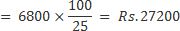## Profit and Loss For SBI PO Set – 4

1) The owner of a watch shop charges his customer 22% more than the cost price. If a customer paid Rs. 8,906 for a wrist watchthen what was the cost price of the wrist watch?

a) Rs. 7,300

b) Rs. 7,500

c) Rs. 7,700

d) Rs. 7,900

e) None of these

a) Rs. 7,300

Explanation

Let the cost price be x

x+22% of x=8906

122x=890600

x=7300.

2) What will be the cost of 14 kg of rice bought at Rs. 38 per kg, 25 kg of sugar bought at Rs. 19 per kg and 9 kg of salt bought at Rs. 23 per kg?

a) Rs. 1214

b) Rs. 1241

c) Rs. 2114

d) Rs. 1244

e) None of these

a) Rs. 1214

Explanation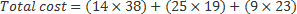=Rs.1214

3) Mohan sold an item for Rs. 4,510 and incurred a loss of 45%. At what price should he have sold the item to have gained a profit of 45%?

a) Rs. 10,900

b) Rs. 12,620

c) Rs. 11,890

d) Cannot be determined

c) Rs. 11,890

Explanation

C.P=x, Loss%=45% , S.P=4510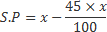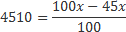x=8200

Profit 45%, then selling price=8200+45% 8200

=8200+3690

=Rs.11890

4) The cost price of an item is two-third of its selling price. What is the gain/loss percent on that item?

a) 45

b) 50

c) 35

d) 54

e) None of these

Explanation

cost price equal to two-third of selling price

So, cost price =2, Selling price=3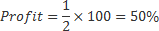5) Abhijit purchased a TV set for Rs. 18,000 and a DVD player for Rs. 4,000. He sold both the items together for Rs. 26,400. How much percent profit did he make?

a) 15%

b) 16 2/3%

c) 20%

d) 18 1/3%

e) None of these

c) 20%

Explanation

C.P of (TV+DVD)=18000+4000=22000

S.P of (TV+DVD)=26400

Profit=sp-cp=4400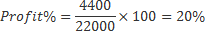6) Meena bought two fans for Rs. 1200 each. She sold one at a loss of 5% and the other at a profit of 10%. What will be the total profit percent or loss percent?

a) 1.2% loss

b) 1.2% profit

c) 2.5% profit

d) 2.5% loss

e) None of these

c) 2.5% profit

Explanation

Total C.P=2×1200=Rs.2400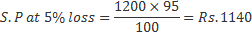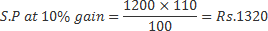Total S.P=1140+1320= Rs.2460

gain=2460-2400=Rs.60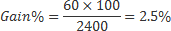7)  A trader sells 40 metres of cloth for Rs. 8,200 at a profit of Rs. 25 per meter of cloth. How much profit will the trader earn on 40 meters of cloth?

a) Rs. 950

b) Rs. 1500

c) Rs. 1,000

d) Rs. 12,00

e) None of these

c) Rs. 1,000

Explanation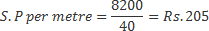C.P per metre= (205-25)=Rs.180

Cost price of 40 metre=40×180=Rs.7200

gain=(8200-7200)=Rs.1000

8) Manoj incurred a loss of 40 percent on selling an article for Rs. 5,700. What was the cost price of the article?

a) Rs. 7,725

b) Rs. 9,080

c) Rs. 8,250

d) Rs. 9,400

e) None of these

e) None of these

Explanation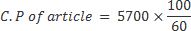= Rs. 9500

9) Sunil sold an antique flower vase to Vinay at 12% more than the cost price. If vinay paid Rs. 17,696 for the vase what was its original cost price?

a) Rs. 15,500

b) Rs. 15,820

c) Rs. 15,800

d) Rs. 15,520

e) None of these

c) Rs. 15,800

Explanation

Cost price = 100/( 100+12) * 176966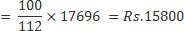10) Prashant incurred a loss of 75 percent on selling an article for Rs. 6,800. What was the cost price of the article?

a) Rs. 27,700

b) Rs. 25,600

c) Rs. 21,250

d) Rs. 29,000

e) None of these